Disclaimer: This is an example of a student written essay.

Any scientific information contained within this essay should not be treated as fact, this content is to be used for educational purposes only and may contain factual inaccuracies or be out of date.

# Multilevel Thresholding According to Histogram

 ✅ Paper Type: Free Essay ✅ Subject: Engineering ✅ Wordcount: 4905 words ✅ Published: 30th Aug 2017

Make Multilevel Thresholding According to Histogram by Cooperative Algorithm based on AFSA and Fuzzy Logic

Image segmentation is a technique which is usually applied in the first step of image analysis and pattern recognition and is an important component of them. This technique is taken into account as one of the most difficult and the most sensitive problems in image analyzing. In this paper, a cooperative algorithm is proposed based on AFSA and k-means. The proposed algorithm is used to make multilevel thresholding for image segmentation according to histogram. In the proposed algorithm, first, artificial fish (AF) perform optimization process in AFSA. After swarm convergence, obtained cluster centers by AFs are used as initial cluster centers of k-means algorithm. After forwarding AFSA’s output to k-means, AFs are reinitialized and performs clustering again. The proposed algorithm is used for segmenting 2 well-known images and obtained results are compared with each other. Experimental results show that segmented images quality by the proposed algorithm is much better than four other tested algorithms.

Keywords: Multilevel Thresholding; Histogram; Cooperative Algorithm; k-means.

Image segmentation is a technique which is usually applied in the first step of image analysis and pattern recognition and is an important component of them. This technique is taken into account as one of the most difficult and the most sensitive problems in image analyzing. In fact, quality of final result of image analysis depends highly on the quality of image segmentation result. In image segmentation process, an image is divided into different regions. Segmentation approaches of mono-color images are with respect to discontinuity and/or similarity of gray level amounts in one region. If the approach performs segmentation based on discontinuities, the image is segmented with respect to abrupt changes on gray level by means of recognizing dots, lines and edges .The purpose of image segmentation approaches is to classify and convert pixels into regions.

If you need assistance with writing your essay, our professional essay writing service is here to help!

Histogram thresholding is one of the techniques, which has been applied extensively in mono-color images segmentation . Generally, images are composed of regions with various gray levels. Therefore, an image’s histogram can consist of some peaks that each of them is related to one region. To separate boundaries of two peaks from each other, a threshold value is considered between valleys of two adjacent peaks. Indeed, histogram thresholding is a famous technique which is looking for peaks and valleys in a histogram . Various clustering algorithms such as k-means  and FCM  have been used for histogram thresholding so far. As a matter of fact, clustering approaches, because of simplicity and effectiveness, belong to the most famous techniques that could be used for natural image segmentation. Applying clustering algorithms in histogram thresholding are such that first colors histogram is built and after that, clustering is done according to color distribution among pixels. One of the clustering methods is to use such swarm intelligence algorithms as particle swarm optimization (PSO) , and artificial fish swarm algorithm (AFSA) . PSO was presented by Kenedy and Eberhart in 1995 . Different versions of this algorithm have been used many times in data clustering . Artificial fish swarm algorithm (AFSA) was presented by Li Xiao Lei in 2002 . This algorithm is a technique based on swarm behaviors that was inspired from social behaviors of fish swarm in nature. AFSA works based on population, random search and behaviorism. This algorithm has been applied on different problems including machine learning [11, 12, 13], PID controlling , image segmentation , data clustering [7, 16] and scheduling . K-means or famous Lloyd algorithm is one of the famous data clustering algorithms . This algorithm is of high convergence rate, but has some weaknesses such as sensitivity to initial values of cluster centers and convergence to local optima. Researchers have tried to remove these weaknesses by hybridizing this algorithm with other algorithms such as swarm intelligence ones [6, 19] and to utilize their advantages. One of these algorithms is KPSO in which first, k-means is performed and after that outcome of k-means is delivered to PSO as a particle . Hence, at the beginning of the algorithm, k-means reaches to a local optimum with its high convergence rate and after that PSO takes the responsibility of increasing the result accuracy and exiting form local optimum.

In this paper, a cooperative algorithm is proposed based on AFSA and k-means. The proposed algorithm is used to make multilevel thresholding for image segmentation according to histogram. In the proposed algorithm, first, artificial fish (AF) perform optimization process in AFSA. After swarm convergence, obtained cluster centers by AFs are used as initial cluster centers of k-means algorithm. After forwarding AFSA’s output to k- means, AFs are reinitialized and performs clustering again. In fact, in the proposed algorithm, AFSA is used for a global search and k-means is used for a local search. The proposed algorithm along with four other algorithms is used for image segmentation on two known images Lenna and Barbara. Efficiency comparison shows that the proposed algorithm has an appropriate and acceptable efficiency.

The remainder of the paper is organized as follows: in sections 2 and 3, standard AFSA and k-means algorithm will be described respectively and in section 4, the proposed algorithm will be presented. Section 5 studies the experiments and analyzes their results and final section concludes the paper.

In water world, fish can find areas that have more foods, which is done with individual or swarm search by fishes. According to this characteristic, artificial fish (AF) model is represented by prey, free-move, and swarm and follow behaviors. AFs search the problem space by those behaviors. The environment, which AF lives in, substantially is solution space and other AF’s domain. Food consistence degree in water area is AFSA objective function. Finally, AFs reach to a point which its food consistence degree is maxima (global optimum).

In artificial fish swarm algorithm, AF perceives external concepts with sense of sight. Current position of AF is shown by vector X=(x 1, x 2,â€¦, x n). The visual is equal to sight field of AF and Xv is a position in visual where the AF wants to go. Then if Xv has better food consistence than current position of AF, it goes one step toward X v which causes change in AF position from X to Xnext , but if the current position of AF is better than X v, it continues searching in its visual area. Food consistence in position X is fitness value of this position and is shown with f(X). The step is equal to maximum length of the movement. The distance between two AFs which are in Xi and Xj positions is shown by Dis ij =||X i-Xj|| (Euclidean distance).

AF model consists of two parts of variables and functions. Variables include X (current AF position), step (maximum length step), visual (sight field), try-number (the maximum test interactions and tries) and crowd factor Î´ (0< Î´<1). Also functions consist of prey behavior, free move behavior, swarm behavior and follow behavior. In each step of optimization process, AF looks for locations with better fitness values in problem search space by performing these four behaviors based on algorithm procedure [10, 14, 17].

The standard k-means algorithm is summarized as follows: Initial position of K cluster centers is determined randomly. The following steps are repeated: a) for each data vector: data vector is allocated to a cluster that its Euclidean distance from its center is smaller than the other cluster’s centers. Distance from cluster center is calculated by Equation (1):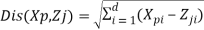(1)

In Equation (1), Xp is data vector p, Zj is the center of cluster j and d is the number of dimensions of data vectors and cluster center vectors. b) After allocating all data to clusters, each of cluster centers is updated by Equation (2):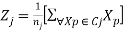(2)

Where, nj is the number of data vectors that belong to cluster j and Cj is a subset of all data vectors which belong to cluster j. The resulted cluster center of Equation (2) is the average vector of data vectors comprising cluster. (a) and (b) steps are iterated until the stopping criterion is satisfied.

In this section, the proposed algorithm is described. In the proposed algorithm, there exists a population of AFSA’s AFs. This population of AFs is initialized randomly in problem space. Each AF consists of K cluster center positions in one dimensional image histogram space. Therefore, search space for AFSA for K cluster centers has K components. Fitness function which AFSA has to minimize is shown in Equation (3).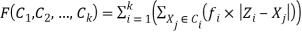(3)

Clustering on histogram is done by Equation (3) based on color distribution between given image’s pixels. The image is divided into K clusters (Ci) according to color attribute by K-1 thresholds. In Equation (3), the distance between color Xj on image histogram and the center of a cluster which it belongs to ( Zi), is multiplied by the frequency of pixels (fj) which have color value Xj on given image. This value is computed for all color values with respect to the center of a cluster which they belong to. Each color becomes the member of a cluster in which their distance from that cluster center is less than other cluster centers. Finally, the obtained results of all clusters are summed with each other. Indeed, Equation (3) calculates sum of intra cluster distances for one dimensional gray scale images, which is one of the most well-known clustering criteria.

For improving obtained results by AFSA, some modifications must do on its structure. The best found position by swarm members so far in AFSA is saved in bulletin and AF which has found it might go even toward worse positions with performing a free-move behavior. Therefore, AFs cannot utilize their best swarm experience for improving the convergence rate because they just save it in bulletin. On the other hand, performing free-move behavior is inevitable for maintaining diversity of the swarm. In this paper, to remove this problem, every AF except best AF can perform free-move behavior. In fact, during execution of the proposed algorithm, this behavior is not performed for the best AF of the swarm at all. Hence, the best found position by the swarm would be the position of the best AF of the swarm. As a result, other members of the swarm can move in the direction of the best found position by executing follow and swarm behaviors.

The purpose of designing the proposed algorithm is to take advantages of both AFSA and k-means algorithms and remove their weaknesses. K-means is of high convergence rate, but it’s very sensitive to initializing the cluster centers and in the case of selecting inappropriate initial cluster centers, it could converge to a local optimum. AFSA can pass local optima to some extent but cannot guarantee reaching to global optima. However, AFSA’s computational complexity for optimization process is much more than k-means. How the proposed algorithm functions remove weaknesses of these two algorithms and apply their advantages is as following:

In the proposed algorithm, first, the AFs are initialized in AFSA. Each of AFSA contains K cluster centers (K-1 threshold) which are displaced in the problem space by performing AFSA’s behaviors. AFSA continues to perform until the AFs converge. After convergence of AFSA, best AF’s position including the best cluster centers which have found by AFs so far is considered as the input of k-means. Then, k-means algorithm starts working and while it is not converged, it continues working. Therefore, AFSA searches globally and as far as it can, it passes local optima. After convergence of AFSA’s AFs, its output would have an appropriate initial cluster centers for k-means. Hence, after sending AFSA’s outcome to k-means, this algorithm starts searching locally. Consequently, in the proposed algorithm, global search ability of AFSA has been used and after converging, a great part of optimization process will be given to k-means to utilize high capability of local search of this algorithm and its high convergence rate. Since initial cluster centers for k-means are obtained by AFSA and k-means is used for local search, k-means weakness of sensitivity to initial cluster centers is removed. But, AFSA capability may not be enough for preventing from being trapped in local optima. If this algorithm is trapped in local optima, it cannot present proper initial cluster values to k-means. Thereafter, according to low ability of k-means in passing local optima, the obtained result cannot be acceptable. To raise this problem, after convergence of AFSA, the output of this algorithm is sent to k-means. Simultaneously with starting of k-means, AFSA’s AFs are initialized and start global search again. In fact, in one time of executing the proposed algorithm, AFSA has several times of chance to perform an acceptable global search. It should be noted that in the proposed algorithm, in each time of executing AFSA, AFs just search globally and converge after a short time and k-means undertakes the remaining of optimization process which is local search. Therefore, with respect to low computational complexity of k-means, huge amount of computations for local search is prevented. In the proposed algorithm, it has been tried to utilize this conserved computation load for giving new opportunities to AFSA in order to perform an acceptable global search in at least one of given opportunities to it. Hence, for each execution of global search by AFSA, k-means is also performed once. In the proposed algorithm, to determine the convergence of artificial fish swarm, the difference of obtained results in consecutive iterations of performing the algorithm is used. When particles converge, the obtained results difference in consecutive iterations decreases, so by considering a threshold for the difference between best AF’s fitness values in iterations i and j, it can determine their convergence. In the proposed algorithm, because AFSA and k-means algorithms are performed multiple times, always, it has to save the best found cluster centers by algorithm so far. For this purpose, a blackboard is applied that each time k-means finishes after convergence of AFSA, the obtained result of that will be compared with saved result in blackboard. If obtained cluster centers are better than saved result in blackboard, saved value in blackboard is updated. K- means execution finishes when after two consecutive iterations of its execution, cluster centers wouldn’t be displaced. Pseudo code of the proposed algorithm is represented in Figure (1).

Experiments are done on two known gray scale images, Lenna and Barbara, of sizes 512*512 in Figure (2). In this paper, the well-known criterion of uniformity is used to compare images’ segmentation qualitatively  which is shown in Equation (4)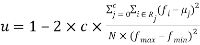(4)

Where, c is the number of thresholds. Rj is the segmented region j. N is the total number of pixels in the given image, fi shows the gray level of pixel I, Âµi is the mean gray level of pixels in jth region, finally, fmin and fmax are the minimum and maximum gray level of pixels in the given image, respectively. Usually, uÏµ[0, 1] and larger amount for u declares that the thresholds are specified with better quality on the histogram.

Proposed Algorithm:

1:for each AFi

2:initialize xi

3:Endfor

4:Blackboard = arg [min F(Xi)]

5:Repeat

6:for each AFi

7:Perform Swarm Behavior on Xi(t) and Compute Xi,swarm

8:Perform Follow Behavior on Xi(i) and Compute Xi,follow

9:if F(Xi,swarm) â‰¥ F(Xi,follow)

10:then Xi(t+1)= Xi,follow

11:Else

12:Xi(t+1)= Xi,swarm

13:Endif

14:Endfor

15:if swarm is converged

16:then Execute k-means on XBest-AF until stopping criterion of k-means is met

17:Endif

18:if F(Xk-means) â‰¤ F(Blackboard)

19:then Blackboard = Xk-means

20:reinitialize AFSA

21:Endif

22:until stopping criterion is met

Figure (1): Pseudo code of proposed algorithm.

The proposed algorithm along with standard AFSA, PSO algorithm, hybrid algorithm called KPSO , and k-means is used to segment two images, Lenna and Barbara. PSO and KPSO parameters are adjusted according to , and for k-means, initializing Forgy method is applied . AFSA parameters and are adjusted according to . AFSA settings in the proposed algorithm are the same as . With respect to various experiments, if fitness value relating to Best AF is less than 0.1 in 3 iterations, it means that artificial fish swarm is converged. The following results are obtained from 50 times repeated experiments. Figure (3) shows segmented images, Lenna and Barbara, by the proposed algorithm with 5 and 3 thresholds.Figure 2: Orginal gray level Lenna (left) and Barbara (right) images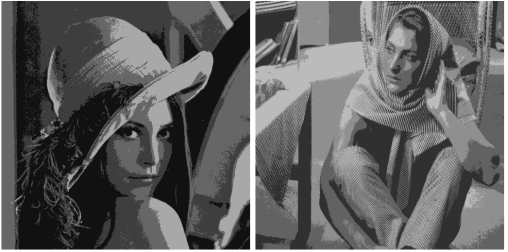Figure 3: The thresholded images of Lenna and Barbara using 5, and 2-level thresholds, from top to bottom.

Average uniformity obtained from 5 algorithms on two images with thresholds 2, 3, 4 and 5 are shown in Table (1). As it is observed in Table (1), obtained results from the proposed algorithm is better than the other algorithms for all cases. AFSA algorithm has the worst result for all cases because of low ability in local search. K-means algorithm has found better results than AFSA because of high capability of k-means in local search. The reason for superiority of k-means to AFSA is the problem space property in histogram clustering. In fact, because of low dimensions of problem space in this environment, local search ability is of greater importance than global search ability. Also, it can reduce k-means weakness of sensitivity to initial values by means of one of the initializing methods of k-means like Forgy. Thereafter, with respect to considerable superiority of k-mean’s local search ability in contrast to AFSA, k-means results are better than AFSA’s.

TABLE I: Comparison of uniformity for the five Algorithms

 Image T AFSA K-means PSO KPSO Proposed method Lenna 2 0.9138 0.9634 0.9730 0.9728 0.9775 3 0.9361 0.9749 0.9781 0.9783 0.9795 4 0.9495 0.9762 0.9816 0.9811 0.9826 5 0.9517 0.9804 0.9835 0.9834 0.9838 Barbara 2 0.9758 0.9761 0.9765 0.9768 0.9781 3 0.9783 0.9802 0.9808 0.9805 0.9820 4 0.9797 0.9834 0.9843 0.9851 0.9862 5 0.9822 0.9849 0.9855 0.9850 0.9884

Obtained results from PSO are better than k-means in all cases and it’s because of global search ability superiority of PSO to k-means. Moreover, in PSO, there’s a trade-off between global search and local search abilities  and PSO also can perform a proper local search beside an acceptable global search. KPSO results are better than k-means results for all cases because after executing k-means in this algorithm, PSO algorithm is performed and improves obtained results from k-means. But obtained results from KPSO are not better than PSO for all cases. The reason is that sometimes k-means converges toward a local optimum and obtained result from that is not appropriate. Therefore, PSO is responsible for taking out the result from local optimum; however, it sometimes may not be successful. Indeed, improper result of k-means causes fast convergence of particles to local optimum. Obtained results from the proposed algorithm are better than other algorithms in all cases. The reason is usage of strategies which have been used for global search in this algorithm. In fact, the proposed algorithm is successful in finding the global optima in most runs and can prevent final result from being trapped in local optima, whereas, this ability is observed less in other algorithms and they cannot guarantee passing local optima. This weakness causes that other algorithms to be of less robustness and not to be able to reach to almost the same results in their various implementations. Also, in the proposed algorithm, k-means algorithm performs local search after finding global optimum region by AFSA. Consequently, with respect to high ability of k-means in local search and taking proper initial cluster centers from AFSA, local search is done well in the proposed algorithm, too. As a result, both k-means and AFSA algorithms abilities are utilized in the proposed algorithm and the weakness of k- means algorithm can’t decrease the algorithm’s efficiency. As it is observed in all algorithms except KPSO, with rising up the number of thresholds, uniformity amount is improved. In KPSO, since the weakness of k-means has an undesirable effect on PSO efficiency, obtained results are not stable.

Our academic experts are ready and waiting to assist with any writing project you may have. From simple essay plans, through to full dissertations, you can guarantee we have a service perfectly matched to your needs.

In this paper, a new cooperative algorithm based on artificial fish swarm algorithm and k-means was proposed for image segmentation with respect to multi-level thresholding. In the proposed algorithm, AFSA performs global search and k-means is responsible for local search. The process of the proposed algorithm is such that the robustness and ability of preventing from being trapped in local optimums is improved. The proposed algorithm along with four other algorithms is used for segmenting 2 well-known images and obtained results are compared with each other. Experimental results show that segmented images quality by the proposed algorithm is much better than four other tested algorithms.

 R. C. Gonzalez, and R. E. Woods, “Digital image processing,” In: Pearson Education India, Fifth Indian reprint, 2000.

 S. Arora, J. Acharya, A. Verma., and K. Panigrahi, “Multilevel thresholding for image segmentation through a fast statistical recursive algorithm,” In: Journal on Pattern Recognition Letters 29, pp. 119–125, 2008.

 Maitra. M, A. Chatterjee, “A hybrid cooperative-comprehensive learning based PSO algorithm for image segmentation using multilevel thresholding,” In: Journal on Expert System with applications 34, pp. 1341-1350, 2008.

 M. Mignote, “Segmentation by fusion of histogram-based k-means clusters in different color spaces,” In: IEEE Transactions on Image Processing, 2008.

 X. Yang, W. Zhao, Y. Chen, and X. Fang, “Image segmentation with a fuzzy clustering algorithm based on Ant-Tree,” In: Journal of Signal Processing 88, pp. 2453-2462, 2008.

 Y. T. Kao, E. Zahara, and I. W. Kao, “A hybridized approach to data clustering,” In: Journal on Expert System with Applications 34, pp. 1754-1762, 2008.

 D. Yazdani, S. Golyari, and M. R. Meybodi, “A new hybrid approach for data clustering,” In: 5th International Symposium on Telecommunication (IST) , pp. 932–937, Tehran, 2010.

 J. Kennedy, and R. C. Eberhart, “Particle swarm optimization,” In: IEEE International Conference on Neural Networks, 4, pp. 1942– 1948, Perth, 1995.

 A. A. A. Esmin, D. L. Pereira, and F. Araujo, “Study of different approach to clustering data by using the particle swarm optimization algorithm,” In: IEEE Congress on Evolutionary Computation, pp. 1817–1822, Hong Kong, 2008.

 L. X. Li, Z. J. Shao, and J. X. Qian, “An optimizing method based on autonomous animate: fish swarm algorithm,” In: Proceeding of System Engineering Theory and Practice, pp. 32-38, 2002.

 D. Yazdani, S. Golyari, and M. R. Meybodi, “A new hybrid algorithm for optimization based on artificial fish swarm algorithm and cellular learning automata,” In: 5th International Symposium on Telecommunication (IST), pp. 932-937, Tehran, 2010.

 D. Yazdani, A. N. Toosi, and M. R. Meybodi, “Fuzzy adaptive artificial fish swarm algorithm,” In: 23 th Australian Conference on Artificial Intelligent, pp. 334-343, Adelaide, 2010.

 J. Hu, X. Zeng, and J. Xiao, “Artificial fish swarm algorithm for function optimization,” In: International Conference on Information Engineering and Computer Science, pp. 1-4, 2010.

 Y. Luo, W. Wei, and S. X. Wang, “The optimization of PID controller parameters based on an improved artificial fish swarm algorithm,” In: 3rd International Workshop on Advanced Computational Intelligence, pp. 328-332, 2010.

 C. X. Li, Z. Ying, S. JunTao, and S. J. Qing, “Method of image segmentation based on fuzzy c-means clustering algorithm and artificial fish swarm algorithm,” In: International Conference on Intelligent Computing and Integrated Systems (ICISS) , pp. 254- 257, Guilin, 2010.

 L. Xiao, “A clustering algorithm based on artificial fish school,” In: 2nd International Conference on Computer Engineering and Technology, pp. 766-769, 2010.

 D. Bing, and D. Wen, “Scheduling arrival aircrafts on multi- runway based on an improved artificial fish swarm algorithm,” In: International Conference on Computational and Information Sciences, pp. 499-502, 2010.

 J. A. Hartigan, “An overview of clustering algorithms,” In: New York: John Wiley & Sons , 1975.

 C. Y. Tsai, and I. W. Kao, “Particle swarm optimization with selective particle regeneration for data clustering,” In: Journal of Expert Systems with Applications 38, pp. 6565–6576, 2011.

 D. W. der Merwe, and A. P. Engelbrecht, “Data clustering using particle swarm optimization,” In: Congress on Evolutionary Computation, pp. 215-220, 2003.

 E. Forgy, “Cluster analysis of multivariate data: efficiency vs. interpretability of classification,” In: Biometrics 21, pp. 768, 1965

View all

## DMCA / Removal Request

If you are the original writer of this essay and no longer wish to have your work published on UKEssays.com then please:

Related Services

Prices from

QAR553

Approximate costs for:

• 1000 words
• 7 day deliveryHumanity University

Dedicated to your worth and value as a human being!

Related Lectures